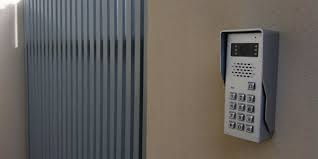# It All Started Nice and Easy...

Probability Level 3In Troll D.C., the capital of United Troll States, there was a man who thought he could answer any problem in the world. So, one day, he was visited by a troll called Sam. Sam told the man: "the intercom on the outside of my math teacher's appartment is broken. On the intercom are the numbers 0 to 9 inclusive and the BAR key. One, to pass through, may enter any 2 numbers, followed by the BAR key, and then any other 4 numbers. One can press the same key again and again. The number of combinations which will allow one to enter are N. Find the number of trailing zeros of N! (N factorial) and call this number A.

Then, find the sum of all roots of the equation $2(A+2)x^4+32x^3+Ax^2-(A+37)x+49=0$. Take the reciprocal of this number to be n. Then find the first 3 significant figures of the infinite sum $1/(2^n) +1/(2^n+1)+ 1/(2^n+2)$... This 3 digit number is K.

Imagine you have a ball, which you throw at angle of $30 ^\circ$ to the horizontal at speed K. Find the time taken for this ball to touch the ground again, assuming that it remains in the same gravitational field and the gravitational force on the ball does not change with distance or height. This is W.

Now imagine that you have W objects laid out in front of you. What is the number of ways to arrange them in a row, knowing that two of them are exactly identical and cannot be differentiated. The number length of this number is H.

How many squares can be drawn on a $H \times H$ chessboard?" The man sat deep in thought for 5 minutes. Then he said: "the number of squares that can be drawn are the number of stupid problems that you have posed".

The troll leaped with glee, for the man had answered incorrectly. He had not taken into account this very problem! The man had got the answer wrong by one!

How many stupid problems has the troll posed, excluding this one? This is an entry in the Troll King Contest.

×